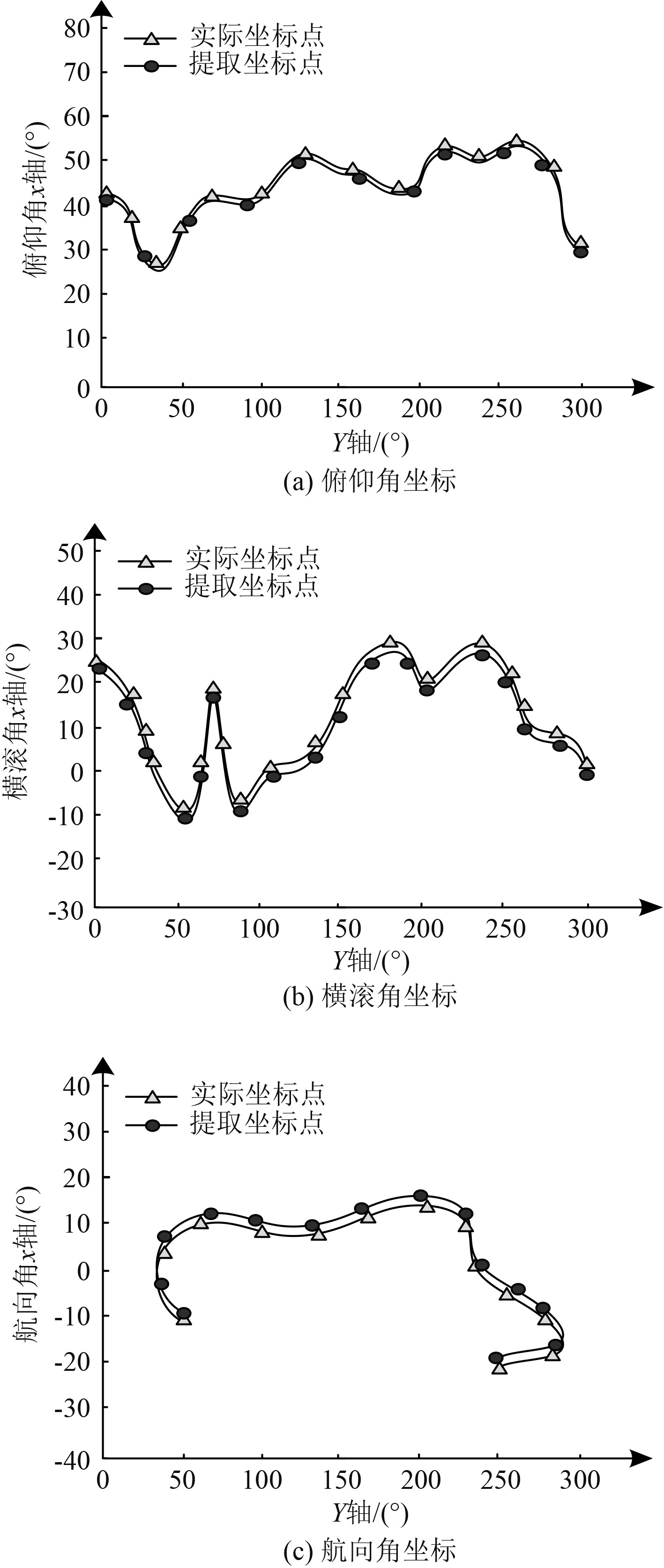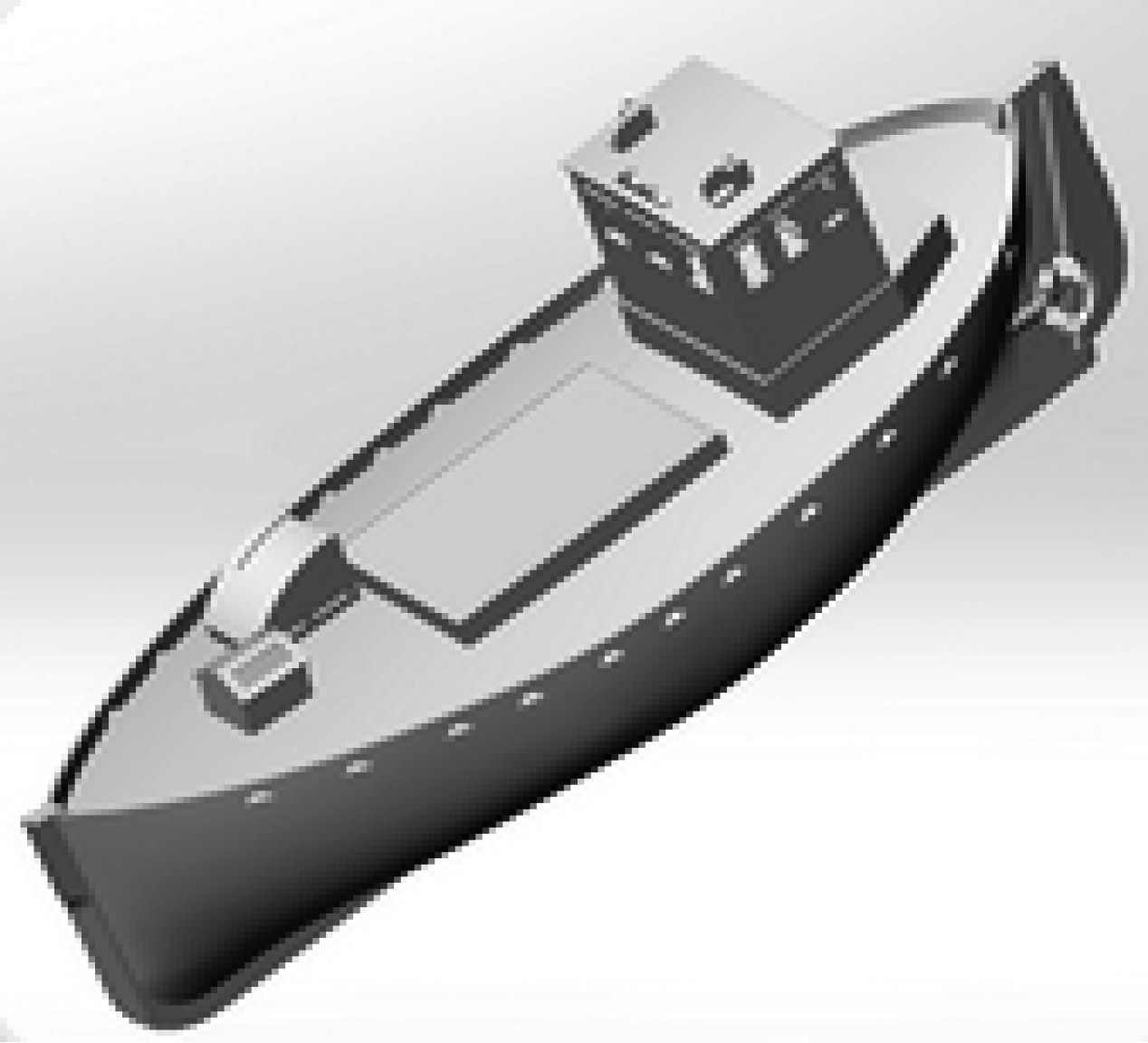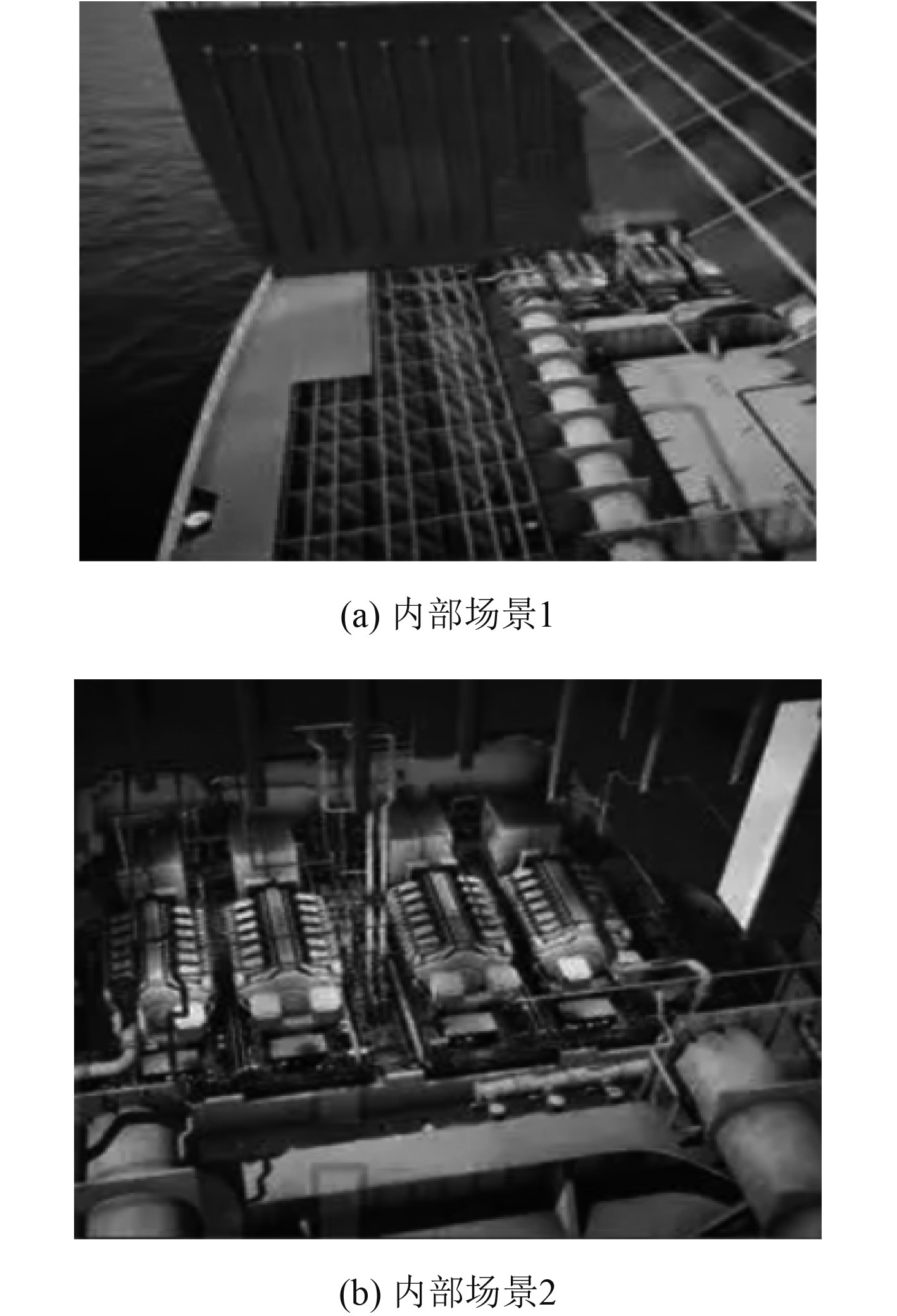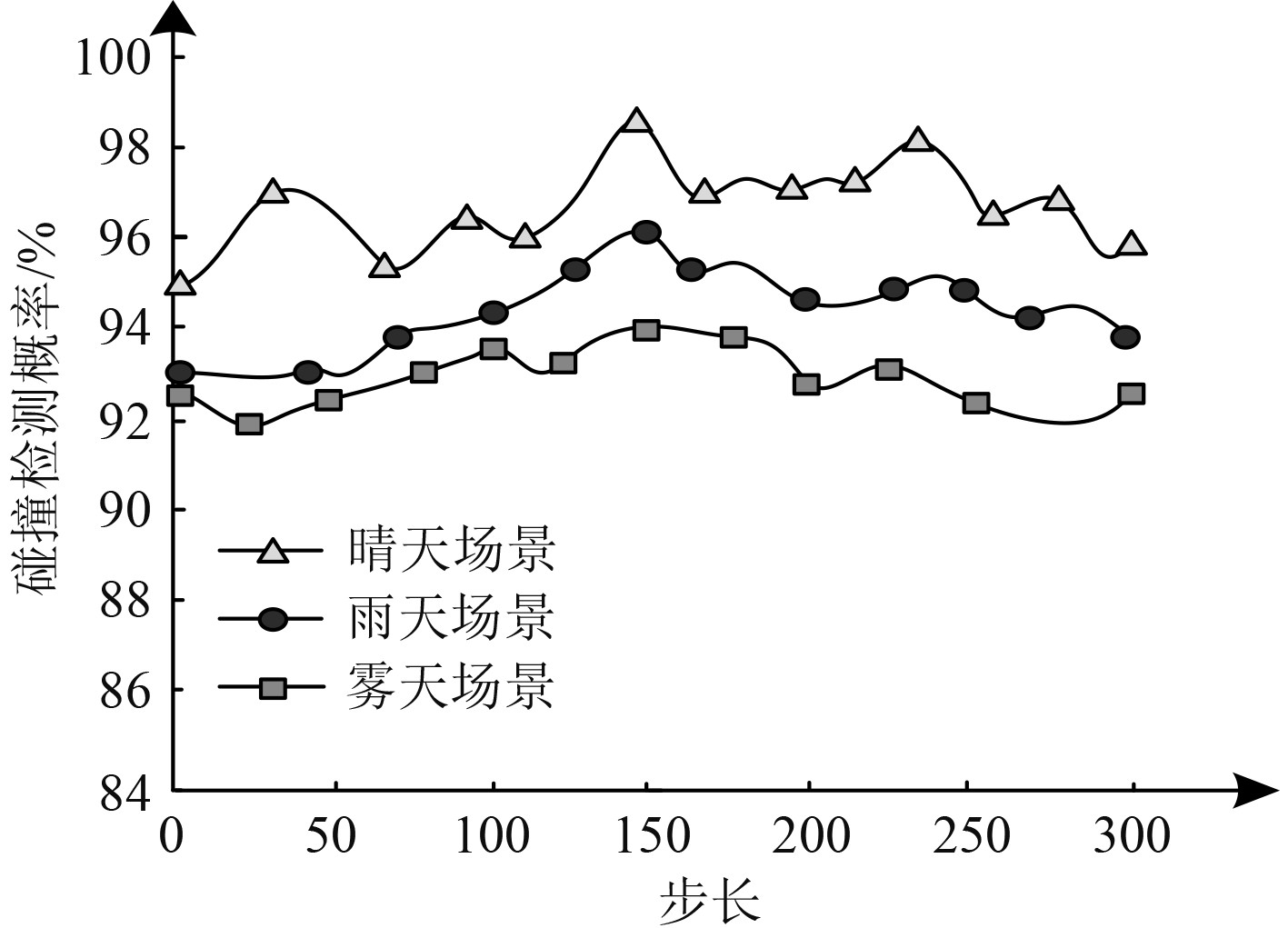﻿ 基于虚拟现实技术的船舶三维建模
 舰船科学技术2022, Vol. 44Issue (9): 40-43    DOI: 10.3404/j.issn.1672-7649.2022.09.008PDF

1. 西南交通大学 信息科学与技术学院，四川 成都 610031;
2. 四川托普信息技术职业学院 计算机系，四川 成都 611743

Research on ship 3D modeling design based on virtual reality technology
ZHANG Li1,2
1. School of Information Science and Technology, Southwest Jiaotong University, Chengdu 610031, China;
2. Computer Science, Sichuan TOP IT Vocational Institute, Chengdu 611743, China
Abstract: Using virtual reality technology to simulate the state of ship three-dimensional model in different scenes can analyze whether the ship itself maintains safe operation in real time. Therefore, a research on ship three-dimensional modeling design based on virtual reality technology is proposed. The CATIA method is used to build the ship 3D model, and AutoCAD is introduced to extract the ship 3D model parameters to obtain the comprehensive ship waterline and angular coordinates, so as to complete the ship 3D model design; Import the ship 3D model into the virtual reality software unity 3D, design the scene object in the software, design the scene roaming, complete the multi scene analysis of the ship, and realize the collision detection of the ship by using the collision detection algorithm based on particle swarm optimization and spatial decomposition hierarchical bounding box (FDH). The method can extract the ship's internal roll angle and pitch angle under different scenarios with an accuracy of more than 92%, and can always detect the ship's internal roll angle through the experiment.
Key words: virtual reality     ship 3D modeling     collision detection     scene roaming     scene object     Unity 3D
0 引　言

CATIA属于当前应用较广的建模技术，通常应用于船舶、机械等多种领域[1-3]，通过该技术进行建模，可以为用户提供较强的二次开发接口[4-5]，能够很好地利用在船舶三维建模中。对船舶三维模型进行虚拟现实场景模拟，能够及时发现船舶运行过程中会出现的故障。时光志等通过虚拟现实技术仿真船舶系统，但该方法构建的船舶三维模型与理想数值相差较大。景乾峰等通过虚拟现实系统构造船舶数字孪生框架，但该方法无法实现船舶多种场景漫游模拟。

1 基于虚拟现实现技术的船舶三维建模方法 1.1 基于CATIA船舶三维建模 1.1.1 构建传统船舶三维模型

1.1.2 基于VB的参数化建模

1)参数提取

2)建立模型

1.2 基于虚拟现实技术的船舶三维建模方案 1.2.1 场景漫游功能设计

1)场景对象分析

2)场景漫游的实现

1.2.2 粒子群与空间分解层次包围盒(FDH)的碰撞检测算法

1)最优化问题

 $\min \sigma = f\left( x \right),$ (1)
 ${\rm{vs.t}}.X \in O = \left\{ {X\left| {{g_i}\left( X \right) \leqslant 0,\;i = 1,\cdots,m} \right.} \right\} 。$ (2)

2) 粒子群算法

 ${H_i}\left( {t + 1} \right) = \left\{ \begin{gathered} {H_i}\left( t \right),f\left( {{X_i}\left( {t + 1} \right)} \right) \geqslant f\left( {{H_i}\left( t \right)} \right)，\hfill \\ {X_i}\left( {t + 1} \right),f\left( {{X_i}\left( {t + 1} \right)} \right) < f\left( {{H_i}\left( t \right)} \right) \hfill 。\end{gathered} \right.$ (3)

 $\begin{split} {H_g}\left( t \right) \in& \left\{ {{H_0}\left( t \right),{H_1}\left( t \right),...,{H_s}\left( t \right)} \right\}\left| {f\left( {{H_g}\left( t \right)} \right)} \right. = \\ & \min \left\{ {f\left( {{H_0}\left( t \right)} \right),{f_1}\left( {H\left( t \right)} \right),\cdots ,f\left( {{H_s}\left( t \right)} \right)} \right\} 。\end{split}$ (4)

 $\begin{split} {v_{ij}}\left( {t + 1} \right) =& {v_{ij}}\left( t \right) + {c_1}{r_{1j}}\left( t \right)\left( {{h_{ij}}\left( t \right) - {x_{ij}}\left( t \right)} \right)+\\ &{c_2}{r_{2j}}\left( t \right)\left( {{h_{gj}}\left( t \right) - {x_{ij}}\left( t \right)} \right) ，\end{split}$ (5)
 ${x_{ij}}\left( {t + 1} \right) = {x_{ij}}\left( t \right) + {v_{ij}}\left( {t + 1} \right)$ (6)

2 实验结果与分析图 1 船舶三维模型内部场景漫游图 Fig. 1 Roaming diagram of internal scene of ship 3D model图 2 船舶三维模型结构图 Fig. 2 Structure diagram of ship 3D model图 3 船舶三维模型内部场景漫游图 Fig. 3 Roaming diagram of internal scene of ship 3D model表 1 船舶建模数据分析 Tab.1 Analysis of ship modeling data图 4 碰撞检测概率分析 Fig. 4 Collision detection probability analysis
3 结　语

  吴乐, 张倩, 单忠德, 等. 面向船舶分段流水线的多工位协同作业建模技术[J]. 船舶工程, 2020, 42(2): 126-130.  陈立家, 刘锭坤, 田延飞, 等. 面向船舶操纵模拟器的内河水流三维建模与仿真[J]. 武汉理工大学学报(交通科学与工程版), 2020, 44(4): 634-639.  何丽丝, 曹荣, 王德禹. 面向送审的船体结构三维模型转化数据技术研究[J]. 中国舰船研究, 2021, 16(5): 206-215.  孙晓雪, 王胜正, 殷非, 等. 智能船舶远程辅助驾驶3D场景构建关键技术研究[J]. 中国舰船研究, 2020, 15(S1): 173-179.  孙佳文, 任鸿翔, 肖方兵, 等. 基于虚拟现实的整船仿真训练平台[J]. 系统仿真学报, 2021, 33(9): 2243-2251.  时光志, 周毅, 李萌, 等. 基于虚拟现实技术的LNG船舶仿真系统[J]. 船海工程, 2021, 50(3): 25-28+33. DOI:10.3963/j.issn.1671-7953.2021.03.007  景乾峰, 神和龙, 尹勇. 一种基于虚拟现实系统的船舶数字孪生框架[J]. 北京交通大学学报, 2020, 44(5): 117-124.  唐能, 刘永珍, 胡志贤, 等. 基于三维体验平台的模型质量管控架构设计研究[J]. 船舶工程, 2020, 42(S1): 149-152+229.